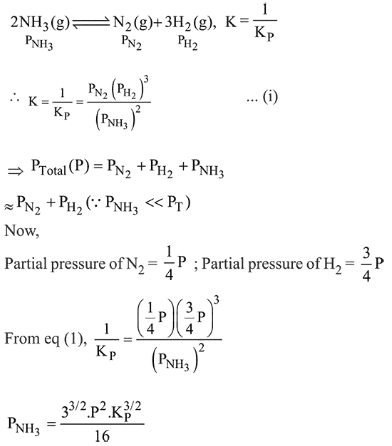# Consider the reactionQuestion:

Consider the reaction $\mathrm{N}_{2}(\mathrm{~g})+3 \mathrm{H}_{2}(\mathrm{~g}) \rightleftharpoons 2 \mathrm{NH}_{3}(\mathrm{~g})$

The equilibrium constant of the above reaction is $\mathrm{K}_{\mathrm{P}}$.If pure ammonia is left to dissociate, the partial pressure of ammonia at equilibrium is given by (Assume that $\mathrm{P}_{\mathrm{NH}_{3}}<<\mathrm{P}_{\text {total }}$ at equilibrium $)$

1. $\frac{3^{3 / 2} K_{P}^{1 / 2} P^{2}}{16}$

2. $\frac{\mathrm{K}_{\mathrm{P}}^{1 / 2} \mathrm{P}^{2}}{16}$

3. $\frac{\mathrm{K}_{\mathrm{P}}^{1 / 2} \mathrm{P}^{2}}{4}$

4. $\frac{3^{3 / 2} K_{P}^{1 / 2} P^{2}}{4}$

Correct Option: 1

Solution: